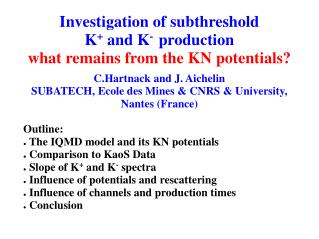DownloadDownload PresentationInvestigation of subthreshold K + and K - production what remains from the KN potentials?

# Investigation of subthreshold K + and K - production what remains from the KN potentials?

Download Presentation## Investigation of subthreshold K + and K - production what remains from the KN potentials?

- - - - - - - - - - - - - - - - - - - - - - - - - - - E N D - - - - - - - - - - - - - - - - - - - - - - - - - - -
##### Presentation Transcript

1. C.Hartnack and J. Aichelin SUBATECH, Ecole des Mines & CNRS & University, Nantes (France) Outline: The IQMD model and its KN potentials Comparison to KaoS Data Slope of K+ and K- spectra Influence of potentials and rescattering Influence of channels and production times Conclusion Investigation of subthreshold K+ and K- productionwhat remains from the KN potentials?

2. Semiclassical microscopic model on an event by event basis Full time-evolution of each event allows for a view inside the reaction Includes nucleons, deltas , pions with their isospin degrees of freedom Nuclear eos, Coulomb, asymmetry, Yukawa and momentum dep. pot. Virtual treatment of strange particles allows for high statistics KN optical potential The IQMD model

3. Parametrisation :RMF calculations of J. Schaffner-Bielich KN optical potential K+pot K- pot. Attention: K-production process via hyperons may involve K+ and K- potentials for K- production K- N pot

4. Calculations in good agreement with data by A.Foerster KN potential penalizes K+ and K- production Comparison to KaoS DATA

5. KaoS claims different slopes of K+ and K- but measures only limited momentum range K+ from IQMD show different slopes at low and high p Comparison of slopes for K+ and K- 4π

6. No influence of K+ potentials on the slope Rescattering influences high energy tail Influence of potential and rescattering

7. Only K+ potential influences the K- slope: reduced production of hyperons K- potential effect only at very low energies Influence of potential on K- spectra

8. K+ are produced in the high density phase while surviving K- stem from later times Analysis of the production time Definition of 3 time windows early t< 8fm/c mid 8<t<16fm/c late t> 16fm/c

9. Strong contribution of early times Similar time profile for all channels Time windows for K+

10. Slope depends on production time Different channels show up at different times Time windows for K- spectra

11. K+ : no strong difference in slopes K- : Different slopes for π–Y and B-Y Contribution of different channels

12. K+ spectra show different slopes at low and high momenta KN-potentials show little effects on slope of K+ K- spectra are only slightly influenced by K- potentials B-Y and π-Y induced K-reactions: Appear at different times Show different slopes Consequently: the experimental slope allows to get information on the production channels but not on the KN potentials Importance of the reaction dynamics for understanding the slopes of the spectra Conclusion

13. (or stuff that you will only see if you really ask for it) Appendix • K+ spectra, centrality dependence • K- spectra, centrality dependence • K- spectra, influence of absorption • cos(J) K+ to K-, K+ potential and rescattering • cos(J) K- potential and absorption • cos(J) K+ time-window, channels, centrality • cos(J) K- time-window, channels, centrality • K+ K- azimuthal j distributions at midrapidity • K+ K- azimuthal distributions: potential • K+ K- azimuthal distributions: rescattering • Souvenirs of SQM 2001

14. Centrality dependence K+ spectra

15. Centrality dependence K- spectra

16. Suppression of absorption recovers many K- produced in early times Influence especially at low momenta Influence of K- absorption

17. K+ cos(J) distributions

18. K- cos(J) distributions

19. K+ cos(J) distributions

20. K- cos(J) distributions

21. K+&K- azimuthal j distributions

22. K+ & K- azimuthal dist.: potential

23. K+ & K- azimuthal dist.: rescattering

24. Souvenirs from SQM 2001

25. Influence of KN potentials